|  home   |  introducton  | part 1  |  part 2  |  part 3  |®  Sergeev Ju.S. Full version in Russian

ARITHMETIC EXCERCISES
in the SOLAR SYSTEM

On the basis of hypothesis about Solar system formating from endo-Galactic Vortex, stated by ┼.╠. Trunaev, who have acquainted the author of the present work with his manuscript in 1991 during personal contact , the results of attempt to apply its main rules to some calculations at the Solar system actually and at planets satellite systems are below stated.

According to this hypothesis, the Solar system was formed from a Galactic Vortex fragment , evolved further in independent vortex structure, in which under gravitation influence in struggle with centrifugal forces the pre-substance of the Vortex was involved in centripetal movement on logarithmic spiral lines which, as is known, have following view: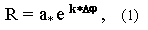where R - radius-vector in any point,
Ó - radius-vector in a reference point ,
e - basis of the natural logarithms,
k - ctg α , angle between the radius-vector and tangent,
φ - angle of turning radius-vector R from the reference radius-vector a.

At the end of pre-planet development phase the pre-Solar Vortex (further - Vortex) had got flat vortex structure, in centre part of which more than 99 % of its weight was already concentrated. The other part of substance was concentrated in two different size spiral branches.

While the alignment of gravitational (centripetal ) and caused by orbital movement centrifugal forces occured, the ruptures of both branches took place, thus peripheral part from each gap place of branches was isolated and further self-organized into affiliated second order vortex.

The centre of newly formed ( affiliated ) vortex , located on the axis of rotation, acquires the centre of weights function , gradually strengthening it by using of additional substance inflow "on a spiral " and, at the same time, moves around the central part of Vortex on circular * orbit with radius, determined as the distance from gap point up to the centre of Solar Vortex rotation. On the same way the subsequent gaps of  Vortex occured .

* actually orbits of their moving around Vortex rotation centre slightly differ from circular. The reason is - gravitational influence of other second order vortexes, separated from the Vortex . This influence is not great but, however, results to some elleptical form of the orbit, which is characterized by value of eccentricity .

Since the reason of all Vortex gaps is uniform, it is natural to suppose, that these gaps occured evenly, periodically in any equal angular intervals Δφ , in that case, from the formula (1) the equation may be written in common view for distances, on which they ( the gaps ) occured; these distances correspond to orbits radiuses in perihelion of affiliated second order vortex , then transformed in planets: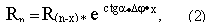where n - orbit ordinal number ( including asteroid zone ).

According to literary data angle a of our Galaxy vortex spiral, in space near Sun , is about 70░. Accepting, as variant, that the pre-Solar Vortex had just the same angle a directly before ruptures appearance and, further second order vortex formation, accor-ding to the ratio of actual outer planets orbits radiuses , corresponding to distances from gap point of the Vortex to its centre, let's determine now at the first approximation the average for whole Vortex Δφ - angle of dividing it by periodic gaps, under which the planets orbits radiuses would change in accordance with the law of logarithmic spiral (2) with α = 70░, while their number reduce. Calculating, we shall consider the assumption stated earlier that the so-called " asteroid zone ", rotating on fifth from Sun regular orbit, traces the destroyed fifth planet ( Phaeton) orbit. Therefore for this calculation we shall consider the serial numbers of planets orbits after Mars one unit larger, than planet numbers today. Let's then make this calculation between Mercury (╣ 1) and Neptune ( ╣ 9) orbits (we shall not take Pluto into account for now because of distinction of its orbit ).

Having decided the equation (2) relatively Δφ and having substituted there the values of these planets orbits radiuses in perihelion, we shall find, that

Δφ = π / 2 .

Let's replace value of e and constant for given spiral ctg α and Δφ, entering additional concept " coefficient of system" Z :

Z = e ctg α Ę Δφ          (3),

then      Z = ų Rn : R(n-x)                  (4)

Now we shall calculate according to formula (4) factor Z of Solar system for interval between ninth (Neptune ) and sixth ( Jupiter ) Vortex gaps,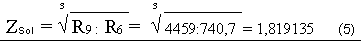and, having decided a equation (3) relatively angle a , we shall obtain more exact value of angle α for pre-Solar Vortex logarithmic spiral: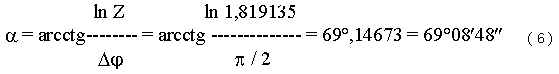Let's analyse whole series of relations values for radiuses of adjacent orbits for all Solar system planets. For the beginning, while try to obtain regularities, we shall exclude from consideration planets orbits , for which radiuse relation to adjacent one more than on 1,5 standard deviations differ from average. These are Mercury orbit ( 2,34> ( 1,71 + 1,5 X 0,41)) and Pluto one (1,00 < ( 1,71- 1,5 X 0,41)).

The remaining row of values is precisely divided on two ones in accordance with value of adjacent orbits radiuses relation: first - from Venus to Mars (1,40 - 1,37) and second - from Mars to Neptune ( 1,63 - 2,03).

We shall determine now value of Δ 2φ ( angle of dividing the vortex) for next inside from external Vortex circuit ( for planets of "Earth" group ) according to formula (3) through the relation of Mars ( ╣ 4) and Venus ( ╣ 2) orbits radiuses: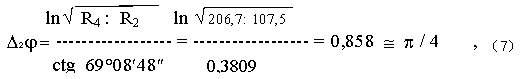( the error in 9 % can be attributed to gravitational interaction of space bodies after their formation, which changed planets orbits ).

Now it is possible to write the formulas for distances, on which regularly in angular interval Δφ = π/2 or Δφ = π/4 its gaps occured; these distances correspond to radiuses of regular ( system ) planets orbits of Solar system, for planets of external and next inside Vortex circuits, accordingly:

Rn = R(n-x) Ę1,8191x       and      Rn = R(n-x) Ę1,8191x/2          (8) & (9)

table 1

 ╣ planet radius of orbit in perigelium, mln.km relation of adjacent orbits radiuses deviation from average Notes 1 2 3 4 5 6 1 Mercury 46 Planets of internal Vortex circuit 2,34 + 0,63 2 Venus 107,5 1,37 - 0,34 3 Earth 147,1 1,40 - 0,31 4 Mars 206,7 2,03 + 0,32 5 (asteroid zone) (420) 1,76 + 0,05 Planets of external Vortex circuit 6 Jupiter 740,7 1,82 + 0,11 7 Saturn 1348 2,03 + 0,32 8 Uranus 2734 1,63 - 0,08 9 Neptune 4459 1,00 - 0,71 10 Pluto 4472 average 1,71 0,41

SATELLITE   SYSTEM  OF  PLANETS

On basis of the assumptions stated by ┼.╠. Trunaev , 1) that satellite system of planets were formed from second order vortex by dividing them to III order vortexes , and 2) that the angle Δφ of dividing for all second order vortexes, as well as for pre-Solar vortex, was for external spiral circuit Δφ = π/2,  for next inside from external circuit Δ2φ= π/4 , we shall calculate parameters of pre-planetary vortexes.

Our method consists the analysis of relations series in pairs for adjacent satellite orbits for each of planet satellite systems, and on the basis of it the calculation average for external and internal intervals of a system. Thus we based on the assumption about equal deviding periodicity of pre-vortexes and consequently, in case of significant deviations each of these relations from average, we offer to explain it in some cases by availability of additional regular orbits, which are "unoccupied" with satellites.

On some planetocentric orbits 2 satellites rotate. Also the pairs of satellites exist, which orbits are so close that we shell consider them as one. These are so-called satellites-antipodes. If to assume, that satellites, which being today in such pairs, were formed from III order vortex as the result of simultaneous gaps in two branches, which, as is known, are there in any vortex, then relation of adjacent orbits radiuses in pairs will be saved identical for all satellites both one and other branches [ fig. 1 ].

As the result of some inequality of satellites-antipodes orbits radiuses and, as the consequence, inequality of orbital speeds, one of satellites overtakes another on close orbit, at small distances gravitational attraction forces begin to influence and as the result of satellites collision the destruction both of them occurs . Thus the wreck material appears, which is "separated" upon the orbit, because of some speeds differences each wreck , forming rings (Uranus , Saturn, Jupiter) like asteroid zones around the Sun. Also separate large wrecks - asteroids appear, some of which continues moving on the same orbit, but other leave initial regular orbit and can be found on irregular orbits, including in satellite system of other planet. Such interpretation of rings availability around planets is probable because of the middles of these rings concur satisfactory with calculated regular satellites orbits .

In accordance with the relation of satellites orbits radiuses each of planets, possessing today satellite system, under the formulas (4) and (6) we shall find factor (Z) for system and angle α (angle of vortex logarithmic spiral) for each pre-planetary vortex:

- for pre-Jupiterian - through orbits radiuses of satellites Io( ╣ 5) and Kallisto(╣ 8);

- for pre-Saturnian - through orbits radiuses of satellites Titan(╣13) and Mimas( ╣ 7)

having assumed thus, that in view of anomalous large relation value of Titan orbit radius to that of next after it Reja, between their orbits should be another regular orbit, which we have conditionally named Ra;

- for pre- Uranian - through orbits radiuses of satellites Oberon(╣ 16) and Umbriel ( ╣ 12), as well as in pre-Saturn system, having assumed under the similar reason the availability of additional regular satellite orbit between orbits known satellites Umbriel (╣12) and Titania( ╣10) , which we have conditionally named Vera;

- for pre-Neptunian - through orbits radiuses of satellites Protey (╣ 8) and Despina(╣ 3), having assumed on similar reasons the availability of two regular satellites orbits between orbits of Protey( ╣ 8 ) and Larissa (╣ 5).

( Since for internal vortex circuit angle of dividing it (Δφ = π ż 4) twice less, than for external (π /2), then the relation of next orbits radiuses is in accordance with formula (9) equal Z 1/2 ).

The parameters calculated thus for all considered pre-planetary vortexes - angle α of logarithmic spiral each vortex and calculation factor Z of satellite ( planetary ) system are indicated in table 2.

table 2

Parameters of pre-Solar and pre-planetary
vortexes and satellite ( planetary ) systems

 The name of vortex Angle α of logarithmic spiral vortex Calculation factor Z of satellite system 1 2 3 4 1 pre-Solar 69░08′48″ 1,8191 2 pre-Jupiterian 72░22′55″ 1,6468 3 pre-Saturnian 75░04′07″ 1,5203 4 pre-Uranian 79░56′50″ 1,3211 5 pre-Neptunian 84░08′14″ 1,1750

Having constructed the graph of factors Z dependence for planets satellites systems from numbers of regular heliocentrical orbits, one can see, that the graph approachs to asimptote on interval from sixth (Saturn ) - to eighth ( Neptune ) regular heliocentrical orbits , that enables, extrapolating this dependence over the Neptune orbit , under linear regression equation, the number of a regular orbit to be determined and, thus, the distance, at which factor Z for next after Neptune satellite system would be equal 1, can be determined too. It would correspond to angle α hipothetical pre-vortex logarithmic spiral to be equal 90░, and so Solar Vortex couldn'tist" the next second order vortex, because at this value of angle α the spiral degenerates in a circle.

sol. bound.orb. = Ņrb.sat.+(ZsÓt - 1):(ZsÓt - Z nept) : 2 = 9,0 ;               (10)

So, the orbit ╣ 9 is boundary for formating of Solar system planets , and it's radius , then, it is possible to consider as the limit of the Solar system:

R sol. bound.orb. =R9 = R8 ? Zsol..= 4459 mln.km ? 1,8191 = 8,1mlrd.km         (11)

If to assume, that the sizes of other satellite systems depend only on weight of their central object, then the limits of any satellite system can be calculated through the limit of the Solar system and through the relation between the planet weight and the Sun one:

m plant.
R plant. bound.orb = R sol. bound.orb --------------- ;                   (12)
M sol.

The limits of satellite systems, calculated thus, are indicated in table 3.

table 3

 calculated limit of The last regular system satellite ╣ satellite system satellite system, mln. km the name factual orbit radius, mln. km 1 2 3 4 5 1 Solar 8100 Neptune 4459 2 Jupiterian 7,7 Kallisto 1,8 3 Saturnian 2,3 Titan 1,2 4 Uranian 0,35 Oberon 0,6 5 Neptunian 0,4 Protey 0,12

As it is clear from table 3, the calculated limits of the so-called planets - giants satellite systems mainly ( except Uranian ) do not contradict to facts.

On the base of calculated limits of satellite systems it becomes clear now, that the Jupiter satellites from Leda to Sinope, Saturn satellites Japet and Feba, Neptune satellite Nereida can not be referred to regular , because of they are over the possibility of their pre-planetary vortex "to twist" the next III order vortex, from which then the satellites could be formed.

On the other hand, at some satellite systems the external regular orbits remain "unoccupied" by satellites beyond last established regular satellite , at Jupiter - 2, at Saturn - 1, and at Neptune -7 orbits.

The calculated limit only for Uranus satellite system is not subject to this established law, as if the weight of planet Uranus would be underestimated not less, than in 583:350=1,6 times. But the reason of it, probably, is uniform with the reason of anomalous direction of its rotation ( " on side " ) and rotation of it's satellites in the plane, almost, perpendicular to ecliptic one and also rotation of Uranus itself around the Sun today on the orbit, distinguished from regular.

As to satellites of other Solar system planets, then, in accordance with the law established above, the satellite systems of planets, if they took place, could not exceed the following sizes, listed in table 4:

table 4

Calculated limits for regular satellites sistems
of Earth group planets and Pluto

 ╣ Satellite system Limit of satellite system thous. km The name of satellite Factual orbit radius, thous. km 1 2 3 4 5 1 of Earth 23 Moon 384,4 2 of Mars 2,5 Fhobos- Deymos 9,38 23,46 3 of Venus 20 - - 4 of Pluto 0,6 Haron 19,64

If the Venus, Mercury, Earth and Mars had their satellite systems, then they have lost them long time ago during interaction with planets-antipodes. In accordance with perfomed calculated limits of satellite systems it becomes clear, in particular, that today's planets satellites of Earth group - the Moon, Fobos and Deymos are not regular satellites, but were formed differently. Insignificant eccentricity and small angles of their orbits inclination permit to state the only possible explanation of their origin, from the view point of hypothesis described above: the Moon, and probably unified satellite (Deymos + Fobos = DeFo ), later destroyed, were formed on opposite branch of Solar Vortex as planets-antipodes, of Earth and Mars accordingly.

The Pluto satellite Haron is not regular as well, and the place of its formation and the reason of its today's presence on Pluto-centrical orbit need to be searched as yet.

Conclusions:

1. All satellite systems of planets, as well as the Solar system, are formed from two- branch pre-planetary (pre-Solar) vortexes.

2. Series of radiuses values satellites (planets ) regular orbits each of satellites ( Solar ) # systems represent geometrical progressions, denominators of which ( the factors of system ) in accordance with the rule decrease from 1,8191 ( external circuit of pre-Solar spiral ) up to 1,175 ( external circuit of pre-Neptune ) while orbits become farther away Solar centre.

3. Angle α of pre-planetary vortexes logarithmic spirals in accordance with the rule increase from 69░,as a round value,( pre-Solar ) up to 84░ ( pre-Neptunian) while the vortexes become farther from centre of pre-Solar one.

4. At each satellite system there is the limit, described by boundary values of main parameters (α =90░, Z=1 ), over which only irregular satellites rotate, formed not on occupied by them today orbit ( the Moon, Fobos, Deymos, Haron, Triton and other are those).

5. The rings from dust and wrecks around planets, having the similarity (in compound and in commonality of structure) with so-called "asteroid zone" around the Sun , represent the destruction product of satellites-antipodes pairs formed originally on opposite branches of pre-planetary vortexes spirals as well.

6. On ōunoccupied" (by satellites ) regular planet-centrical orbits the opening of new satellites is possible. The list of these orbits, established on the basis of calculations offered here, on which satellites or their wrecks are not found yet , is perfomed in table 5.

table 5
Calculated regular planet-centrical orbits on which

 Belonging to Calculated approximate parameters of possible satellite The name of regular orbit satellite system regular orbit period ofrotation possible satellite, diameter weight ╣ place radius,thous. km Earth days thous. km 23g* 10 1 2 3 4 5 6 7 8 Jupiterian 10 after Kallisto 3101 35 Jupiterian 11 after Kallisto 5108 75 S XXI Saturnian 6 orb.of Mimas 185 0,94 0,15 0,2 S XX 7 orb. of Encelad 229 1,37 0,15 0,2 ( RÓ) 11 Rea - Titan 804 8,5 1 100 13 after Titan 1858 30 U13 Uranian 2 ring - Kordelia 47 0,3 0,015 (Genius) 7 Pek - Miranda 110 0,8 0,1 0,2 (Vera) 11 Umbriel - Titania 334 5,5 1 100 N IX Neptunian 5 Larissa - Protey 85 0,8 0,1 0,2 N X 6 Larissa - Protey 100 1,0 0,1 0,2 8 after Protey 138 1,4 9 after Protey 162 1,8 10 after Protey 191 2,3 11 after Protey 224 2,9 12 after Protey 263 3,7 13 after Protey 309 4,8 14 after Protey 364 6,1

 |  home |  introduction |  part 1  |  part 2  |  part 3  |